# Experimental and theoretical study on thermal decomposition of methyl butanoate behind reflected shock waves†

A. Parandaman, M. Balaganesh and B. Rajakumar*
Department of Chemistry, Indian Institute of Technology Madras, Chennai-600036, India. E-mail: rajakumar@iitm.ac.in

Received 22nd August 2015 , Accepted 2nd October 2015

First published on 5th October 2015

## Abstract

Thermal decomposition of methyl butanoate (MB) diluted in argon was studied behind reflected shock waves in the temperature range of 1229–1427 K using a single pulse shock tube (SPST). The post shock mixtures were analyzed quantitatively using gas chromatography (GC) and qualitatively using Fourier-transform infrared (FTIR) spectroscopy. Methane (CH4), ethylene (C2H4), and acetylene (C2H2) were the major decomposition products. The minor products are ethane (C2H6), propylene (C3H6), 1,3-butadiene (C4H6) and methyl acrylate (C4H6O2). The obtained first order rate coefficient for the decomposition of MB is ktotal (1229–1427 K) = (3.08 ± 1.11) × 1012exp(−(53.6 kcal mol−1 ± 4.7)/RT) s−1, and for the formation of C2H4, the rate coefficient was found to be kethylene (1229–1427 K) = (7.92 ± 2.72) × 109exp(−(47.6 kcal mol−1 ± 4.5)/RT) s−1. Theoretical kinetic calculations were also performed for the unimolecular hydrogen transfer reactions using canonical variational transition state theory (CVT) with small-curvature tunneling (SCT) corrections. The temperature dependent rate coefficients for the overall reaction were computed in the temperature range of 500–2500 K, and were used to derive the Arrhenius expression: ktheorytotal (500–2500 K) = (9.05 ± 1.91) × 1013exp(−(70.7 kcal mol−1 ± 2.0)/RT) s−1. A reaction scheme containing 39 species and 66 elementary reactions was proposed to simulate the reactant and product concentrations over the temperature range of 1229–1427 K. The agreement between the experimental results and the model prediction for all the species is observed to be good. The decomposition of MB happens mostly via intramolecular hydrogen transfer than C–C bond and C–O bond fission. The majority of these intramolecular hydrogen transfer reactions are lower energy barrier reactions than the homolytic bond fission reactions.

## 1. Introduction

Solid, liquid, or gaseous biofuels are derived from renewable biological sources. Biomass can be converted to high value energy sources including ethanol, biodiesel, methanol, hydrogen, or methane.1,2 They are considered as alternative fuels for the transport sector and are expected to reduce our dependence on petroleum. In addition, biofuels minimize the effects on the climate because they produce fewer greenhouse gases than gasoline or diesel.3,4 Biodiesel is the most widely used biofuel. Typical biodiesel consists of mixtures of saturated and unsaturated methyl esters containing carbon chains of 16 or more atoms in length that are generally derived from animal fats and vegetable oils5,6 (soybean oil in U.S. and rapeseed oil in Europe). Although methyl butanoate (MB) does not have larger molecular weight like a typical biodiesel, it has the essential chemical structural features, namely the R–(C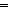O)–O–CH3 structure (where R is an alkyl group). Hence, the resultant reaction mechanism is smaller and will be of more manageable size than that of a larger hydrocarbon.7 The reaction rate coefficients for larger methyl esters are primarily based on the kinetic parameters of smaller methyl esters (e.g., methyl butanoate).8,9 Therefore, accurate knowledge of the kinetic parameters for such smaller methyl esters is essential in the development of the detailed mechanism of biodiesels for practical purposes.

The combustion chemistry of lower methyl esters has gained a focus of attention for the past one and half decade.10 MB has been chosen as a surrogate for biodiesel fuels, and various theoretical and experimental studies on this molecule have been reported in the literature.11–14 Fisher et al.15 developed the first complete chemical kinetic mechanisms for the oxidation of methyl formate and MB. However, they have validated their mechanism with the available experimental data in temperature range of 550–675 K. Hakka et al.16 developed detailed chemical kinetic mechanism for the oxidation of MB, based on their shock tube and a jet-stirred reactor experimental data. Similarly, Dooley et al.17 developed a detailed mechanism for the oxidation of MB and it was tested with their shock tube and rapid compression reactor data. They have reported the ignition delay times as well. A review by Lai et al.18 summarizes the research to date on biodiesel molecules, including MB. Recently Ali and Violi19 studied unimolecular decomposition pathways (C–C, C–O bond fissions and hydrogen migrations) of MB and the corresponding rate constants, using ab initio methods in combination with transition state theory. In addition, the rate coefficients for the unimolecular elimination channels were computed using the master equation approach, in their studies. Overall, various studies on MB pyrolysis and oxidation were carried out with appropriate kinetic mechanisms. However, most of these studies have paid attention only on oxidation of MB.

In the present investigation, thermal decomposition of MB was studied experimentally for the first time in the temperature range of 1229–1427 K behind the reflected shock waves. The complete degradation products were followed and appropriate mechanism for the decomposition is suggested in this study. In order to further understand the experimental measurements, we also report the rate coefficients for hydrogen transfer reactions in thermal decomposition of MB using canonical variational transition state theory (CVT) coupled with the hybrid meta density functional M06-2X with 6-31+G(d,p) basis set in broad temperature range of 500–2500 K. This study provides a complete picture of the major decomposition pathways of MB and the results can be used to improve the accuracy and completeness of the MB kinetic mechanism.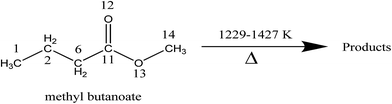## 2. Experimental

The thermal decomposition of MB was studied behind the reflected shock waves in a 50.8 mm i.d. single pulse shock tube (SPST). The driver and driven section lengths are 1290 mm and 3440 mm respectively. The details of the experimental setup used in this work have been given elsewhere.20 A 10 liter dump tank was connected to the driven section at 45° angle near the diaphragm station in order to prevent reflection of transmitted shock waves. The driver section was separated from the driven section by an aluminum diaphragm of various thicknesses depending upon the desired shock strength. Three pressure transducers are mounted towards the end of the driven section. The mounted pressure transducers were used to measure the shock velocity and thereby to calculate both the primary and reflected shock temperatures. The pressure transducer, which is mounted closest to the end flange, was used to record the pressure trace and reaction time, in the reaction zone. A typical pressure trace recorded using a piezoelectric pressure transducer mounted near the end of the driven section is shown in Fig. 1. The arrival of both the primary and the reflected shock waves, and the reaction time are marked in the pressure trace. The constant voltage in the “reaction time” zone is the direct evidence for the nearly constant temperature condition in the carried out experiments. Shock velocities were calculated from the time taken for the shock wave to travel between the pressure transducers mounted on the driven section near the end of the shock tube. The reflected shock temperatures calculated by conventional Rankine–Hugoniot relations.21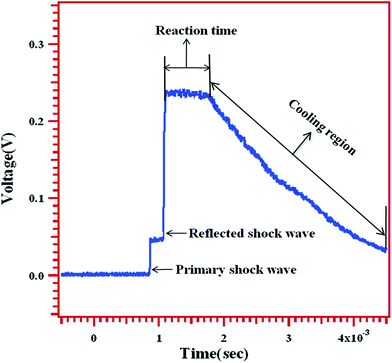Fig. 1 A typical pressure trace recorded by an oscilloscope showing the arrival of primary, reflected shock waves, reaction time and expansion/cooling wave.

The chemical thermometric method22,23 was used in our investigations to get accurate reflected shock temperatures. Reflected shock temperatures were determined from the extent of decomposition of 1,1,1-trifluoroethane (CH3CF3) which was added in small quantities to the reaction mixtures to serve as an internal standard. It decomposes into CH2CF2 + HF is a first order unimolecular reaction, which has a rate coefficient24 of k = 5.71 × 1046(T)−9.341exp(−47073K/T) s−1. The extent of decomposition of the internal standard in a given experiment, χ can be calculated using the below given equation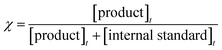where [product]t is product concentration of internal standard at time ‘t’ and [internal standard]t is left out concentration of internal standard at time ‘t’.

Using the calculated, the rate coefficient for the decomposition of the internal standard at the carried out experimental temperature and the reaction time is calculated using the below given relation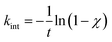The temperature behind the reflected shock wave can now be calculated using the temperature dependent rate coefficient equation for the unimolecular elimination of HF from 1,1,1-trifluoroethane reported by Akira et al.24

The reflected shock temperatures calculated using Mach number (T5-Ms) and internal standard method (1,1,1-trifluoroethane as internal standard) were observed to be differed by ∼5–6% in the studied temperature range. The temperatures calculated using shock speed are higher by ∼5–6%, which is acceptable given the errors in measurement of the speed of the shock, real gas effects and boundary layer effects. The use of chemical thermometric method eliminates most of the inherent uncertainties in the physical properties in these shock tube experiments as determined from the conservation equations. The introduction of the internal standard removes uncertainty in experiments because both the internal standard and target molecule experience the same experimental conditions such as temperature, heating time and reaction pressures. Therefore, the temperatures measured using chemical thermometry method were chosen in our experiments and in subsequent analyses of the data.

The shock tube was pumped down to approximately 1 × 10−6 Torr for two to three times before the experimentation using a diffusion pump, after making the shock tube rich in argon environment. Reaction mixture containing 2% of MB and 0.2% of diluted CH3CF3 were prepared manometrically and diluted further with argon gas to a desired pressure. The reaction mixtures were loaded in the sample compartment of the shock tube, which is at the end of the driven section separated using a ball valve. While the sample compartment was filled with the sample and argon, the rest of the driven section was filled only with argon to a little higher pressure (about 10–15 Torr), to avoid the back diffusion of the sample when the ball valve is opened just before generating a shock wave. The shock waves were generated by rupturing a pre-scored aluminum diaphragm by loading desired pressure of ultra high pure helium in the driver section. After the experiment, the samples were directly transferred to the online six port gas sampling valve (having a constant volume loop of 0.5 ml) of the gas chromatograph (Agilent Technologies 6890N) for quantitative analyses through a pre evacuated gas transfer line. The transfer lines were purged with the post shocked mixture for sufficient time to flush out any gases that were present. Neither the reactant (MB) nor any of the products (methane, ethane, ethylene, propylene, acetylene, 1,3-butadiene and methyl acrylate) were condensed on the walls as their vapour pressures are very high at room temperature. In addition, the partial pressures of all these compounds in the reaction mixture were much less when compared with their vapour pressures. Therefore, the transfer lines were not heated to avoid any condensation. Lighter compounds (C1–C4) were more efficiently separated in a 2 m long packed alumina column. Heavier gas components were separated in 2 m long Porapak-Q column. The oven temperature was programmed from 35 °C to 150 °C in both the cases. Nitrogen was used as a carrier gas in the analyses. The sensitivity of the flame ionization detector (FID) towards all the reactants and products were calibrated over a known range of concentrations. The concentration/mole fraction of left-out reactant and other products were calculated using the known sensitivity factors obtained in the calibration and the areas under each peak. Parallelly, the samples were analyzed qualitatively in FTIR spectrometer (Bruker's VERTEX 70).

## 3. Materials and chemicals

MB (99%), methyl acrylate (99%) purchased from Sigma Aldrich and CH3CF3 (99% purity) were from SynQuest Laboratories were used in these experiments. MB was further purified by several cycles of freeze–pump–thaw method. Chromatograms of procured samples did not show any products that appeared in the post shock mixtures. Methane (99.5%), ethylene (99.5%), ethane (99.5%), propylene (99.5%), 1,3-butadiene (99.5%) and high purity helium gas (99.995%) were purchased from Praxair and were used as such in our experiments without further purification.

## 4. Computational

### 4.1. Quantum chemical calculations

All electronic structure calculations were carried out using the Gaussian 09 program suite.25 The geometries of all stationary points on potential energy surface of MB decomposition pathways were optimized with M06-2X level of theory with recommended 6-31+G(d,p) basis set.26,27 The geometries of the reactant, transition states and products at M06-2X/6-31+G(d,p) level of theory are presented in Fig. 2. This functional was verified to be performed well in predicting geometries and vibrational frequencies.28–30 This functional is also reported to produce excellent results for reaction kinetics of gas phase reactions and was reported that the calculated rate coefficients in general are very good in agreement with the experimentally measured rate coefficients.28–30 The normal modes of reactants, transition states and products were viewed in Gauss View.31 All the reactants and products were confirmed with zero imaginary frequencies (NImag = 0), and transition states were confirmed with one imaginary frequency (NImag = 1). The minimum energy path (MEP) was obtained by intrinsic reaction coordinate32 (IRC) calculations using M06-2X/6-31+G(d,p) to verify that the transition states connect the designated reactants and products. By employing the POLYRATE2008 (ref. 33) GAUSSRATE2009A34 programs, the theoretical rate coefficients were calculated over the wide temperature range of 500–2500 K using the canonical variational transition state theory35–37 (CVT) with small-curvature tunneling38,39 (SCT) method.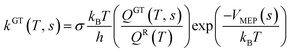kCVT(T) = minskGT(T, s) = kGT[T, SCVT(T)]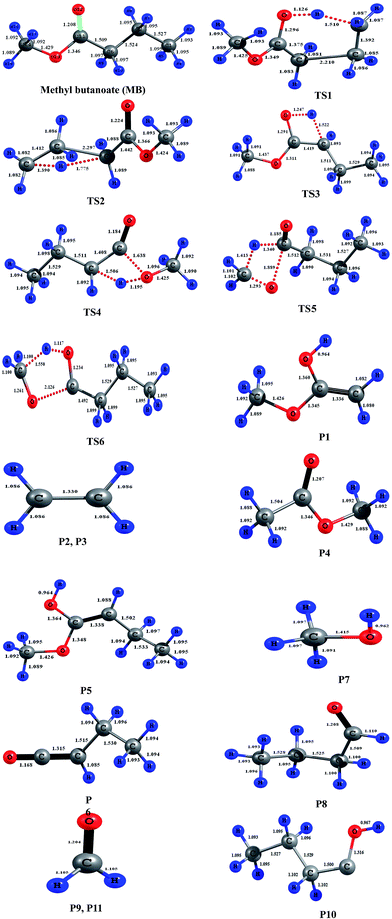Fig. 2 Geometries of the reactant, transition states, and products optimized at the M06-2X/6-31+G(d,p) level of theory. Blue color represents hydrogen and gray color represents carbon, red color represents oxygen in the structures. The bond lengths (Å) given on the structures are obtained at M06-2X/6-31+G(d,p) level of theory.

In these equations, kGT and kCVT are the rate coefficients of generalized and canonical variational transition state theories respectively, ‘s’ is reaction path degeneracy, kB is Boltzmann's constant, h is Planck's constant, T is temperature in Kelvin, SCVT is the reaction coordinate (s) at which canonical variational transition state dividing surface was found. QGT and QR are the partition functions of a generalized TS at ‘s’ and reactants respectively. VMEP(s) is the potential energy of generalized TS at ‘s’. The canonical variational transition state is located by maximizing the free energy of activation with respect to ‘s’.

### 4.2. Electronic structures and energetics

A total of six transition states were identified and optimized. These transition states correspond to six different reactions where intramolecular hydrogen transfer happen, vide infra. The relative energies (ΔE0 in kcal mol−1) obtained for all TSs using different level of theories are summarized in Table 1. The vibrational frequencies and structural parameters of various species involved in the six reaction pathways obtained at the M06-2X/6-31+G(d,p) level of theory are given in the ESI (Tables S-I and S-II). The potential energy surface is shown in Fig. 3 in which all the possible transition states and products are labeled. All the six proposed reactions are pictorially represented along with the corresponding structures in Fig. 4. This figure illustrates the intramolecular hydrogen transfer reactions in thermal decomposition of MB, and the corresponding six reaction channels.
Table 1 Energy barriers (kcal mol−1) for thermal decomposition of methyl butanoate reaction via pathways (R1)–(R6), calculated at M06-2X/6-31+G(d,p)
TSs M06-2X/6-31+G(d,p)
TS1 67.39
TS2 104.76
TS3 70.68
TS4 73.56
TS5 80.77
TS6 104.94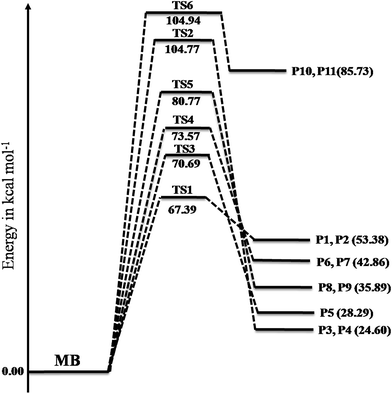Fig. 3 Potential energy surfaces of reaction channels (R1)–(R6) computed using the M06-2X/6-31+G(d,p) level of theory. The energies are given in the units of kcal mol−1.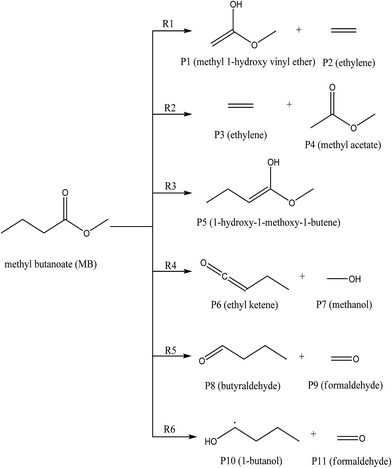Fig. 4 The reaction pathways ((R1)–(R6)) for the unimolecular decomposition of methyl butanoate considered in this study.

The reaction (R1) proceeds through a hydrogen transfer from C1 to O12 through a six-membered transition state TS1, leading to the formation of products P1 (methyl-1-hydroxy vinyl ether) and P2 (ethylene) through a keto–enol isomerization process. In TS1, the breaking C–H, C–C bond lengths and forming O–H bond lengths are increased by about 38%, 45% and 17% when compared to the C–H, C–C bond in MB and O–H bond in product P1 respectively. This pathway is the low barrier pathway with a barrier height of 67.4 kcal mol−1, which is in very good agreement with the values reported earlier in the literature.19,40 The relative energy of the products P1 and P2 is 53.4 kcal mol−1.

In reaction (R2), a hydrogen transfer occurs from C1 to C6 via four membered transition state TS2, followed by the breaking of C2–C6 bond leading to the formation of products P3 (ethylene) and P4 (methyl acetate). In transition state TS2, the breaking C–H, C–C bond lengths and forming C–H bond lengths are increased by about 27%, 51% and 63% when compared to the regular C–H, C–C bond lengths in MB and forming C–H bond length in product P4 respectively. The barrier height (104.8 kcal mol−1) for this reaction is significantly higher compared to those of other reaction pathways (except reaction (R6)). The reported barrier heights for this channel are in good in agreement with the present study. The relative energy of the products P3 and P4 is 25 kcal mol−1.

The hydrogen transfer in reaction (R3) take place from C6 to O12 via transition state TS3. The breaking C–H bond and forming O–H bond lengths are increased by 39% and 29% when compared to the normal C–H bond length in MB and O–H bond length in product P5 (1-hydroxy-1-methoxy-1-butene) respectively. The barrier height for this reaction is 71 kcal mol−1. The barrier height for this reaction is also in good agreement with the one reported by Ali and Violi.19 The reaction energy for the formation of product P5 is 28 kcal mol−1. In reaction (R4), the hydrogen transfer occurs from C6 to O13 through transition state TS4 leading to the formation of products P6 (ethyl ketene) and P7 (methanol), whose relative energy is 28 kcal mol−1, and the barrier energy for the reaction is 73 kcal mol−1. The hydrogen transfer from carbon atom to oxygen atom is less favorable than in the reaction (R3). In TS4, the breaking C–H bond length and forming O–H bond length are increased by 37% and 24% when compared to C–H normal bond length in MB and O–H bond length in product P6.

In reaction (R5), due to intramolecular hydrogen transfer from C14 to C11 via four centered transition state TS5 followed by breaking of C11–O13 bond in MB. The barrier height for this pathway is 81 kcal mol−1. The primary products formed in the reaction are P8 (butyraldehyde) and P9 (formaldehyde) with relative energy 36 kcal mol−1. In TS5, breaking C–H and C–O bond lengths are increased by 29% and 21% when compared to C–H and C–O bond lengths in MB. The formed C–H bond is increased by 28% when compared with the C–H bond length in product P8. Similarly in the case of reaction (R6), the transfer of hydrogen from C14 to O12 through a five membered transition state TS6 leading to the formation of products P10 (1-butanol radical) and P11 (formaldehyde) with relative energy of 86 kcal mol−1. The barrier energy for this reaction pathway is 105 kcal mol−1, which is significantly higher barrier and less possible reaction compared to all other reaction pathways in current study. The breaking C–H, C–O bond lengths are increased by 42% and 58% when compared to C–H, C–O bond lengths in MB. The forming O–H bond length is increase by 16% when compared to O–H bond length in product P10. The relative energy of the products P10 and P11 is 86 kcal mol−1.

The reaction enthalpies and Gibbs's free energies for thermal decomposition of MB were calculated at M06-2X/6-31+G(d,p) to predict the spontaneity. The values of ΔH0298 K (kcal mol−1) and ΔG0298 K (kcal mol−1) are given in Table 2. All studied reaction pathways ((R1)–(R6)) are endothermic and non spontaneous reactions at room temperature. However, these reactions become important with the increase in temperature. Therefore, they are considered in this study, as the working temperatures of this study are in the range of 1229–1427 K.

Table 2 The reaction enthalpies (ΔH0298 K, kcal mol−1), free energies (ΔG0298 K, kcal mol−1) are calculated at M06-2X/6-31+G(d,p) level of theory
Reaction ΔH0 (kcal mol−1) ΔG0 (kcal mol−1)
(R1) 54.12 43.01
(R2) 25.47 13.91
(R3) 28.35 28.26
(R4) 43.76 31.75
(R5) 36.66 25.82
(R6) 86.60 75.48

### 4.3. Kinetic analysis

Rate coefficients for reactions (R1)–(R6) were computed using canonical variational transition state theory with small curvature tunneling (CVT/SCT) in the temperature range of 500–2500 K. The Arrhenius plot for the computed rate coefficients for reaction pathways (R1)–(R6) at M06-2X/6-31+G(d,p) level of theory in the temperature range of 500–2500 K is shown in Fig. 5. The temperature dependent rate coefficients for all the pathways were fit by linear least-squares method and the parameters are tabulated in Table 3. Among all the intramolecular hydrogen transfer reactions of MB, reactions (R1) and (R3) showing higher rate coefficients, and reaction (R6) is found to have lower rate coefficients. To validate our results, we compared the rate coefficients of reactions (R1)–(R5) with the values reported by Ali and Violi19 as shown in Fig. 5 and the agreement between these two studies seems to be very good.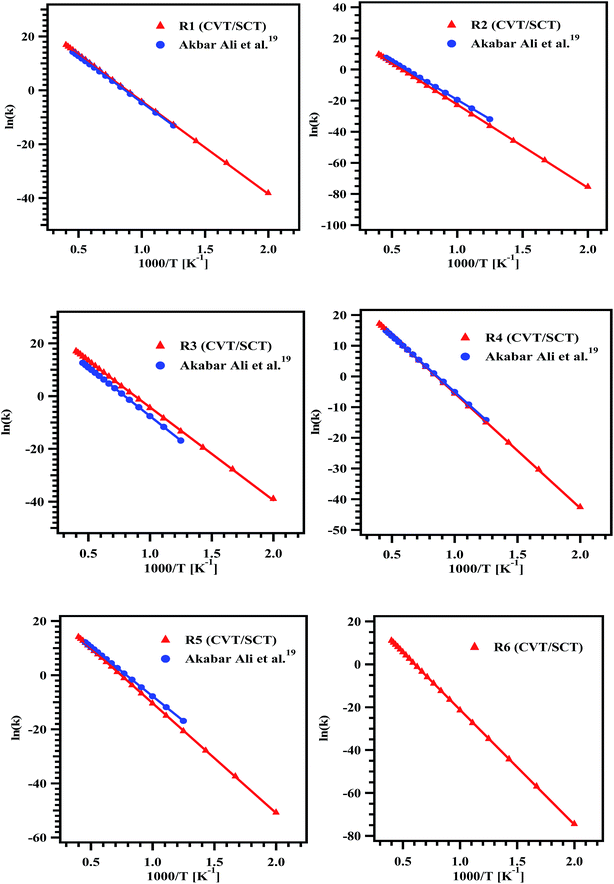Fig. 5 Arrhenius plots for the rate coefficient data obtained for the reaction pathways (R1)–(R6) over the temperature range of 500–2500 K.
Table 3 Arrhenius parameters for thermal decomposition of methyl butanoate via reaction pathways ((R1)–(R6)) in the temperature range of 500–2500 K
Pathway A Ea (kcal mol−1)
(R1) (1.62 ± 0.22) × 1013 68.5 ± 0.3
(R2) (2.51 ± 0.64) × 1013 106.3 ± 0.5
(R3) (2.66 ± 0.50) × 1013 69.9 ± 0.2
(R4) (7.56 ± 0.98) × 1013 74.4 ± 0.3
(R5) (1.36 ± 0.15) × 1013 80.7 ± 0.2
(R6) (3.51 ± 0.50) × 1013 106.4 ± 0.2

The overall rate coefficient for the decomposition of MB was obtained by summing the rate coefficients of individual unimolecular reaction channels at the respective temperatures. The overall rate coefficients are plotted along with the experimentally measured rate coefficients for the complete decomposition of MB in Fig. 10. The data reported by Ali and Violi19 was appended to this plot. To our surprise, the agreement between all these rate coefficients is excellent. Therefore, it can be concluded from our theoretical calculations that, the unimolecular reaction channels ((R1)–(R6)) mostly governs the reaction. The theoretically calculated rate coefficients were used to fit the Arrhenius equation in the studied temperature range using linear least square method. The temperature dependent Arrhenius expression for the title reaction was obtained to be ktheorytotal (500–2500 K) = (9.05 ± 1.91) × 1013exp(−(70.7 kcal mol−1 ± 2.0)/RT) s−1. Also, the computed rate coefficients are in very good agreement with experimentally measured rate coefficients in the temperature range of 1229–1427 K. The rate coefficients computed using the theoretical methods in the present study are for the intramolecular hydrogen transfer reactions, via which the MB decomposes to form majority of the products. However, the rest of the products, methane in particular can be explained only via C–C, C–O and C–H bond scission channels. These channels were not explored theoretically in the present study. The rate coefficients for the rest of all the possible reaction pathways such as C–C, C–O and C–H bond scissions were taken from the literature for the purpose of kinetic simulations, vide infra. Therefore, the theoretically calculated temperature dependent rate coefficient is because of the intramolecular hydrogen transfer reactions alone. However, the temperature dependent rate coefficient deduced using the experimental data is due to all the possible channels. Therefore, the activation energy obtained using theoretical methods is higher when compared with that of the experimentally obtained one. The activation energy can be lowered, if all the possible channels can be optimized using theoretical methods, which is a very difficult task. This may be the main reason for large difference in activation energy for the overall decomposition rate between experiments and theory.

## 5. Results and discussion

To understand the decomposition mechanism and distribution of reaction products, 35 experiments were carried out with gas mixtures containing 2% of MB and 0.2% of diluted CH3CF3 in argon, covering the temperature range of 1229–1427 K. Detailed conditions of each experiment and the normalized yields of products are given in Table 4. The detectable products that were observed in the decomposition of MB and CH3CF3 are methane (CH4), ethane (C2H6), ethylene (C2H4), acetylene (C2H2), 1,1-difluoroethylene (CH2CF2), propylene (C3H6), 1,3-butadiene (C4H6) and methyl acrylate (C4H6O2). The product CH2CF2 originates from the decomposition of CH3CF3 which was used as an internal standard. The other products CH4, C2H6, C2H4, C2H2, C3H6, C4H6, and C4H6O2 are formed from the decomposition of MB. To confirm this, MB alone was decomposed behind the reflected shock waves in the studied temperature range and confirmed the formation of all these products in the GC analyses of the post shock mixture. Typical chromatograms of the post shocked mixture of an experiment carried out at 1378 K are shown in Fig. 6 and 7. The concentration ratios of CH2CF2 and CH3CF3 were used to determine the temperatures behind the reflected shock waves.
Table 4 Experimental conditions and distribution of normalized concentrations of reactant and reaction products in the decomposition of methyl butanoate
S. no. T5 (K) Reaction time (μs) [CH4]t/[C5H10O2]0 [C2H6]t/[C5H10O2]0 [C2H4]t/[C5H10O2]0 [C3H6]t/[C5H10O2]0 [C2H2]t/[C5H10O2]0 [1,3-C4H6]t/[C5H10O2]0 [C5H6O2]t/[C5H10O2]0 [C5H10O2]t/[C5H10O2]0
1 1229 584 0.01230 0.00596 0.01341 0.003466 0.002673 0.000722 0.001233 0.960237
2 1230 457 0.02156 0.00493 0.01552 0.004954 0.008290 0.003264 0.011533 0.929953
3 1232 682 0.02912 0.00590 0.01750 0.006813 0.002353 0.001567 0.002962 0.933780
4 1240 491 0.02156 0.00493 0.01552 0.004954 0.008290 0.003264 0.011533 0.929953
5 1251 744 0.03984 0.00641 0.02536 0.007734 0.005616 0.011417 0.001778 0.901847
6 1260 684 0.05425 0.00615 0.02847 0.008170 0.021269 0.003841 0.005952 0.871905
7 1276 696 0.04037 0.00566 0.03243 0.004986 0.039022 0.002978 0.013582 0.860962
8 1276 580 0.03148 0.00609 0.02610 0.007164 0.008571 0.003572 0.006210 0.910809
9 1277 452 0.03688 0.01124 0.03998 0.007174 0.018230 0.005439 0.002603 0.878450
10 1284 564 0.04505 0.01462 0.03985 0.008070 0.016793 0.007893 0.001058 0.866678
11 1289 818 0.05498 0.01339 0.05212 0.009239 0.029218 0.002986 0.005118 0.832947
12 1295 670 0.04043 0.00571 0.03243 0.004985 0.039018 0.002978 0.013581 0.860867
13 1303 680 0.06134 0.01450 0.05874 0.008736 0.041740 0.002128 0.005042 0.807775
14 1311 774 0.13060 0.01623 0.08207 0.014363 0.137430 0.003521 0.011278 0.604520
15 1319 588 0.05857 0.00901 0.04229 0.012033 0.011907 0.003025 0.009372 0.853791
16 1320 650 0.06874 0.01155 0.05889 0.007763 0.060447 0.002094 0.007695 0.782825
17 1324 540 0.05871 0.00901 0.04228 0.012031 0.011905 0.003024 0.009370 0.853667
18 1325 570 0.03515 0.00684 0.03139 0.005708 0.014977 0.002114 0.006709 0.897117
19 1327 532 0.15271 0.02345 0.10664 0.027993 0.048701 0.005343 0.017624 0.617540
20 1335 600 0.06247 0.01140 0.05861 0.007483 0.062123 0.003128 0.006242 0.788532
21 1340 467 0.05928 0.01050 0.04617 0.010872 0.017819 0.002544 0.009437 0.843381
22 1346 658 0.11629 0.01760 0.07245 0.018736 0.037860 0.001832 0.017728 0.717498
23 1347 640 0.11775 0.02013 0.07506 0.018871 0.037990 0.001838 0.017932 0.710425
24 1366 670 0.12645 0.01510 0.08925 0.016331 0.077262 0.002844 0.012089 0.660673
25 1372 755 0.18682 0.01608 0.10880 0.024061 0.122621 0.003442 0.016185 0.521997
26 1377 774 0.21688 0.02014 0.12235 0.024747 0.240024 0.006843 0.022138 0.346883
27 1377 674 0.24750 0.03855 0.18181 0.037535 0.094566 0.013705 0.029305 0.357029
28 1378 768 0.12010 0.01231 0.07829 0.015856 0.083282 0.004186 0.018941 0.667027
29 1380 619 0.18679 0.02331 0.11317 0.019489 0.192119 0.008741 0.018788 0.437596
30 1396 970 0.21095 0.01908 0.10338 0.018181 0.310251 0.003449 0.004589 0.330111
31 1399 754 0.18839 0.01719 0.11358 0.022306 0.166663 0.004248 0.015199 0.472420
32 1404 808 0.25211 0.02213 0.13493 0.030202 0.279184 0.009899 0.009944 0.261597
33 1406 804 0.32549 0.03765 0.19912 0.028518 0.279981 0.008944 0.007975 0.112323
34 1412 978 0.24215 0.01627 0.12876 0.021409 0.359627 0.007228 0.004809 0.219753
35 1427 908 0.29436 0.02359 0.20773 0.015344 0.360894 0.004949 0.003619 0.089506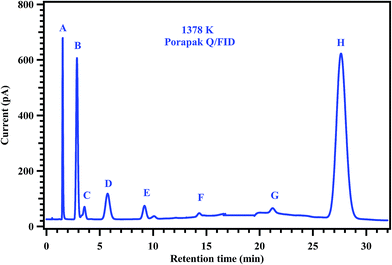Fig. 6 Gas chromatogram showing the products of the post shock mixture of the experiment carried out at 1378 K. The peaks labeled in the chromatogram are (A) methane, (B) ethylene, (C) ethane, (D) propylene, (E) acetylene, (F) 1,3-butadiene, (G) methyl acrylate, and (H) methyl butanoate.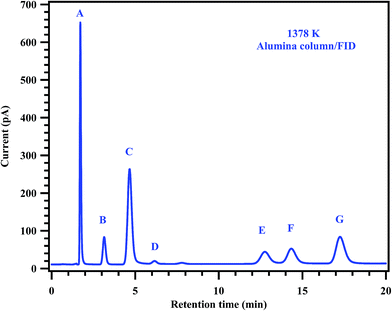Fig. 7 Gas chromatogram showing the products of the post shock mixture of the experiment carried out at 1378 K. The peaks labeled in the chromatogram are (A) methane, (B) ethane, (C) ethylene, (D) 11-DFE, (E) acetylene, (F) 111-TFE and (G) propylene.

FT-IR analyses were carried out to find out the products formed in the post shock mixture, qualitatively. A representative FT-IR spectrum of the post shock mixture of MB in argon, decomposed at 1378 K is shown in Fig. 8. The spectrum shows the presence of MB with a band at 1825 cm−1, and bands in the region 2900–3050 cm−1. The bands in the region 2050–2220 cm−1 show the presence of CO, the bands assigned at 3250–3300 cm−1 and band with high intensity at 750 cm−1 shows the presence of acetylene.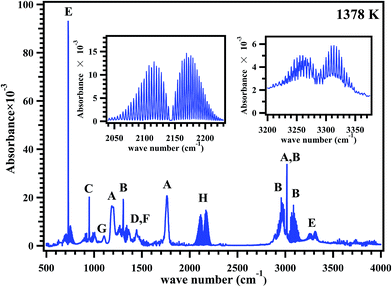Fig. 8 FTIR spectrum of the post shock mixture of methyl butanoate diluted in argon pyrolyzed at 1378 K. All the peaks are assigned to (A) methyl butanoate, (B) methane, (C) ethylene, (D) ethane, (E) acetylene, (F) propylene, (G) methyl acrylate and (H) CO; inset plots shown are the expanded regions for CO and acetylene.

The decay of the reactant and formation of all the products are shown as a function of temperature in Fig. 9 with filled circles. The concentrations of the products except methyl acrylate were observed to be increasing with the temperature. However, the concentration of methyl acrylate was observed to be increasing up to 1400 K and then started decreasing with the temperature. The maximum yields for the products methane, ethane, ethylene, acetylene, propylene, 1,3-butadiene and methyl acrylate were 33%, ∼3%, 21%, 36%, ∼3%, ∼1% and 3% respectively. The product distribution profiles were later compared with the concentration profiles obtained using kinetic simulations, vide infra. Fig. 9a–h shows the comparisons between the concentrations of products based on analyses of post shock mixtures and those from kinetic simulation with the reaction scheme given in Table 5, vide infra. The solid symbols in the figure are experimental points, and the open symbols represent the simulated ones. The agreement between the experimental concentrations and the simulated values are observed to be good.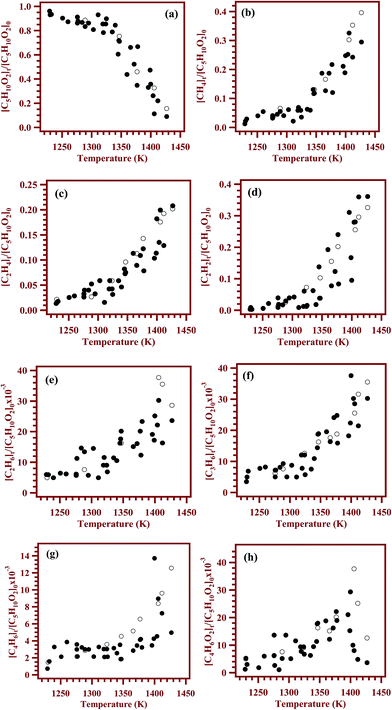Fig. 9 Comparison between the experimentally measured and simulated concentrations of (a) methyl butanoate, (b) methane, (c) ethylene, (d) acetylene, (e) ethane, (f) propylene (g) 1,3-butadiene and (h) methyl acrylate. Filled circles on the plot are experimental concentrations and the open circles are simulated concentrations.
Table 5 Proposed reaction scheme for the decomposition of methyl butanoate with 39 reaction species and 66 elementary reactionsa
S. no. Reaction A n Ea Reference
a Rate expressions are given in the form of k = Aexp(−Ea/RT) and k = ATnexp(−Ea/RT). The units of the rate coefficients are s−1 and cm3 mol−1 s−1 for first and second order reactions respectively. The units for the activation barrier are kcal mol−1.
(R1) MB → C2H4 + CH2C(OH)–O–CH3 1.62 × 1013 0.00 68.46 This work
(R2) MB → CH3–C(O)–O–CH3 + C2H4 2.51 × 1013 0.00 106.30 This work
(R3) MB → CH3–CH2–CHC(OH)–O–CH3 2.66 × 1013 0.00 69.98 This work
(R4) MB → CH3–CH2–CHC(OH)–O–CH3 + CH3–OH 7.56 × 1013 0.00 74.36 This work
(R5) MB → CH3–CH2–CH2–C(O)–H + H–C(O)–H 1.36 × 1013 0.00 80.71 This work
(R6) MB → CH3–CH2–CH2–C(OH) + H–C(O)–H 1.03 × 1014 0.00 106.38 This work
(R7) MB → CH3˙ + CH2˙–CH2–C(O)–O–CH3 5.41 × 1015 −0.19 80.00 19
(R8) MB → CH3–CH2˙ + CH2˙–C(O)–O–CH3 4.03 × 1015 −0.18 77.70 19
(R9) MB → CH3–CH2– CH2˙ + CH3–O–C(O)˙ 2.15 × 1016 −0.25 87.60 19
(R10) MB → CH3–CH2–CH2–C(O)˙ + CH3–O˙ 4.30 × 1015 −0.28 92.50 19
(R11) MB → CH3–CH2–CH2–C(O)–O˙ + CH3˙ 6.58 × 1015 −0.22 83.60 19
(R12) MB + H → CH3–CH2–CH2–C(O)–O–CH2˙ + H2 9.61 × 102 3.34 3.94 42
(R13) MB + H → CH2˙–CH2–CH2–C(O)–O–CH3 + H2 1.50 × 104 2.96 4.29 42
(R14) MB + H → CH3–CH˙–CH2–C(O)–O–CH3 + H2 4.95 × 105 2.47 3.26 42
(R15) MB + H → CH3–CH2–CH˙–C(O)–O–CH3 + H2 3.18 × 104 2.77 2.28 42
(R16) CH3–CH2–CH2–C(O)–O–CH2˙ + H2 → MB + H 2.87 × 103 2.68 11.01 42
(R17) CH2˙–CH2–CH2–C(O)–O–CH3 + H2 → MB + H 2.22 × 101 3.26 9.06 42
(R18) CH3–CH˙–CH2–C(O)–O–CH3 + H2 → MB + H 3.38 × 102 2.83 11.41 42
(R19) CH3–CH2–CH˙–C(O)–O–CH3 + H2 → MB + H 4.71 × 101 3.28 14.92 42
(R20) MB + CH3˙ → CH3–CH2–CH2–C(O)–O–CH2˙ + CH4 2.28 × 10−8 5.88 6.79 42
(R21) MB + CH3˙ → CH2˙–CH2–CH2–C(O)–O–CH3 + CH4 1.50 × 104 2.96 4.29 42
(R22) MB + CH3˙ → CH3–CH˙–CH2–C(O)–O–CH3 + CH4 1.44 × 10−5 4.97 7.18 42
(R23) MB + CH3˙ → CH3–CH2–CH˙–C(O)–O–CH3 + CH4 1.87 × 10−5 4.97 6.06 42
(R24) CH2˙–CH2–CH2–C(O)–O–CH3 + CH4 → MB + CH3˙ 2.22 × 101 3.26 9.06 42
(R25) CH3–CH˙–CH2–C(O)–O–CH3 + CH4 → MB + CH3˙ 4.35 × 10−8 5.59 13.85 42
(R26) CH3–CH2–CH˙–C(O)–O–CH3 + CH4 → MB + CH3˙ 1.24 × 10−7 5.71 17.24 42
(R27) CH3–CH2–CH2–C(O)–O–CH2˙ + CH4 → MB + CH3˙ 3.02 × 10−7 5.48 12.39 42
(R28) CH2˙–CH2–CH2–C(O)–O–CH3 → CH3–CH2–CH2–C(O)–O–CH2˙ 1.34 × 10−8 5.43 15.75 42
(R29) CH2˙–CH2–CH2–C(O)–O–CH3 → CH3–CH2–CH˙–C(O)–O–CH3 1.49 × 10−23 10.03 16.71 42
(R30) CH2˙–CH2–CH2–C(O)–O–CH3 → CH3–CH˙–CH2–C(O)–O–CH3 5.08 × 10−17 8.45 23.69 42
(R31) CH3–CH˙–CH2–C(O)–O–CH3 → CH2˙–CH2–CH2–C(O)–O–CH3 1.05 × 10−17 8.50 26.79 42
(R32) CH3–CH˙–CH2–C(O)–O–CH3 → CH3–CH2–CH˙–C(O)–O–CH3 9.71 × 10−9 5.71 23.82 42
(R33) CH3–CH˙–CH2–C(O)–O–CH3 → CH3–CH2–CH2–C(O)–O–CH2˙ 1.43 × 10−7 5.16 16.82 42
(R34) CH3–CH2–CH˙–C(O)–O–CH3 → CH2˙–CH2–CH2–C(O)–O–CH3 1.33 × 10−23 10.29 24.58 42
(R35) CH3–CH2–CH˙–C(O)–O–CH3 → CH3–CH˙–CH2–C(O)–O–CH3 4.83 × 10−8 5.92 28.60 42
(R36) CH3–CH2–CH˙–C(O)–O–CH3 → CH3–CH2–CH2–C(O)–O–CH2˙ 9.80 × 10−11 6.55 21.75 42
(R37) CH3–CH2–CH2–C(O)–O–CH2˙ → CH3–CH2–CH˙–C(O)–O–CH3 6.62 × 10−10 6.26 15.65 42
(R38) CH3–CH2–CH2–C(O)–O–CH2˙ → CH3–CH˙–CH2–C(O)–O–CH3 2.40 × 10−6 5.07 15.48 42
(R39) CH3–CH2–CH2–C(O)–O–CH2˙ → CH2˙–CH2–CH2–C(O)–O–CH3 4.06 × 10−8 5.40 17.51 42
(R40) CH3–CH˙–CH2–C(O)–O–CH3 → C3H6 + C(O)˙–O–CH3 4.53 × 1012 0.33 34.27 42
(R41) CH3–CH2–CH˙–C(O)–O–CH3 → CH3O˙ + CH3–CH2–CHCO 1.46 × 1012 0.61 53.28 42
(R42) CH3–CH2–CH˙–C(O)–O–CH3 → CH2CH–C(O)–O–CH3 + ˙CH3 1.33 × 1011 0.97 34.88 42
(R43) CH2˙–CH2–C(O)–O–CH3 → CH2CH–C(O)–O–CH3 + H 1.53 × 108 1.57 38.38 42
(R44) CH2CH–C(O)–O–CH3 + H → CH2CH–C(O)–O–CH2˙ + H2 1.42 × 103 3.29 3.98 42
(R45) CH2CH–C(O)–O–CH3 + H → CH2˙–CH2–C(O)–O–CH3 4.18 × 108 1.56 1.70 42
(R46) CH2CH–C(O)–O–CH3 + H → CHCH–C(O)–O–CH3 + H2 2.80 × 106 2.36 12.21 42
(R47) CH2CH–C(O)–O–CH3 + H → CH2C˙–C(O)–O–CH3 + H2 1.98 × 107 2.05 12.16 42
(R48) CH˙CH–C(O)–O–CH3 → C2H2 + C(O)˙–O–CH3 5.76 × 1012 0.82 38.50 42
(R49) CH3–CH2–CH2–C(O)–O–CH2˙ → CH3–CH2–CH2–C(O)˙ + HC(O)H 1.23 × 1013 0.38 36.71 42
(R50) CH2–CH2–CH2–C(O)–O–CH3 → C2H4 + CH2˙–C(O)–O–CH3 5.25 × 1011 0.50 26.59 42
(R51) CH2CH–C(O)–O–CH2˙ → CH2˙–CHCO + H–C(O)–H 2.83 × 1011 0.49 30.00 42
(R52) CH2–CHCO → C2H3˙ + CO 3.47 × 1012 0.63 27.21 42
(R53) CH3–CH2–CH2˙ → C3H6 + H 4.14 × 1012 0.17 35.62 43
(R54) 2CH3˙ → C2H6 6.80 × 1014 −0.60 0.00 44
(R55) 2CH3˙ → C2H4 + H2 9.90 × 1015 0.00 32.98 45
(R56) 2CH3˙ → C2H5˙ + H 2.41 × 1013 0.00 12.87 46
(R57) CH3–O–C(O)–CH2˙ → CH2C(O) + CH3 1.28 × 1012 0.66 49.26 42
(R58) CH2C(O) + H → CO + CH3˙ 7.77 × 108 1.45 2.78 47
(R59) CH3–O–C(O)˙ → CO + CH3–O˙ 8.02 × 1011 0.65 21.12 42
(R60) CH3–O˙ → H–C(O)–H + H 1.87 × 1025 −3.00 24.31 48
(R61) H–C(O)–H + H → CH3–O˙ 1.99 × 108 1.66 1.72 42
(R62) C3H6 + C2H3˙ → C4H6 + CH3 7.23 × 1011 0.00 5.01 49
(R63) C2H3˙ → C2H2 + H 2.00 × 1014 0.00 39.74 50
(R64) CH4 + C3H5˙ → C3H6 + CH3˙ 3.99 × 101 3.40 23.25 49
(R65) C2H4 + H → C2H3˙ + H2 5.24 × 1014 0.00 14.90 50
(R66) C3H6 + C2H5˙ → C2H6 + C3H5˙ 2.23 3.50 6.64 49

The rate coefficients for the overall decomposition of MB were calculated as a first-order rate constant from the relation.

 ktotal = −ln{[MB]t/[MB]0}/t
where [MB]t and [MB]0 are the experimentally quantified concentrations of MB at the end of the reaction time ‘t’ and ‘t = 0’ (initial concentration) respectively. The obtained rate coefficients were used to plot the Arrhenius equation and it is shown in Fig. 10. The data were fit using linear least square method and the obtained rate coefficient for overall decomposition of MB in the entire temperature range is ktotal (1229–1427 K) = (3.08 ± 1.11) × 1012exp(−(53.6 kcal mol−1 ± 4.7)/RT) s−1, where R is expressed in the units of kcal K−1 mol−1. The obtained Arrhenius parameters for the overall decomposition of MB in present experimental and the theoretical study are given in Table 6.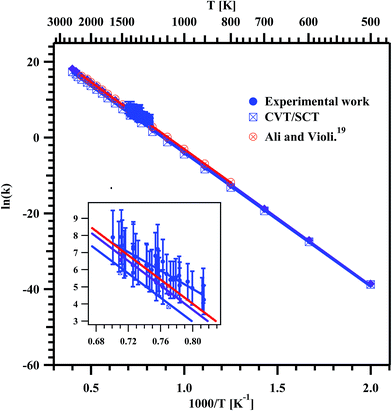Fig. 10 Arrhenius plot for the overall decomposition of methyl butanoate in the temperature range of 1229–1427 K. The obtained temperature dependent rate coefficient for the entire experimental temperature range is ktotal (1229–1427 K) = (3.08 ± 1.11) × 1012exp(−(53.6 kcal mol−1 ± 4.7)/RT) s−1. The insert is the zoom of the data obtained in the present experiments.
Table 6 The present experimental and theoretical rate parameters for total decomposition of methyl butanoate and formation of ethylene in the studied temperature range
Temperature range A (s−1) Ea (kcal mol−1) Reference
Total decomposition of MB
1229–1427 K (3.08 ± 1.11) × 1012 53.6 ± 4.7 This work (experiment)
500–2500 K (9.05 ± 1.91) × 1013 70.7 ± 2.0 This work (CVT/SCT)Ethylene formation from decomposition of MB
1229–1427 K (7.92 ± 2.72) × 109 47.6 ± 4.5 This work (experiment)
500–2500 K (5.52 ± 0.89) × 1012 68.5 ± 1.6 This work (CVT/SCT)

The rate coefficients for the formation of ethylene were computed by using the following relation.41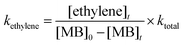where [ethylene]t is the concentration of ethylene at the end of reaction time ‘t’. The experimentally obtained rate coefficients were used to plot the Arrhenius equation and are given in Fig. 11. The data were fit using linear least squares method and the temperature dependent rate coefficient for the formation of ethylene was obtained to be kethylene (1229–1427 K) = (7.92 ± 2.72) × 109exp(−(47.6 kcal mol−1 ± 4.5)/RT) s−1. Fig. 11 shows the measured rate coefficients for the formation of ethylene from the decomposition of MB in the present experimental and theoretical study along with the RRKM/ME study of Ali and Violi.19 The present experimental and theoretical rate parameters for the formation of ethylene in the studied temperature range are given in Table 6. The experimentally measured rate coefficients are very good in agreement with available literature rate coefficients and computed rate coefficients of present study in the experimental temperature range of 1229–1427 K.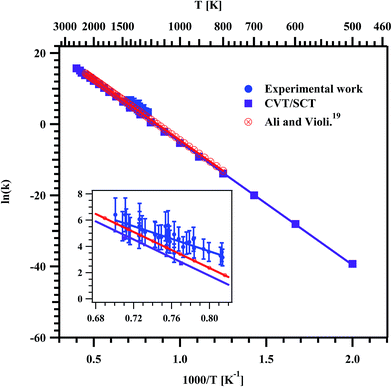Fig. 11 Arrhenius plot for the formation of ethylene in the decomposition of methyl butanoate. The obtained temperature dependent rate coefficient for the entire experimental temperature range is kethylene (1229–1427 K) = (7.92 ± 2.72) × 109exp(−(47.6 kcal mol−1 ± 4.5)/RT) s−1. The insert is the zoom of the data obtained in the present experiments.

The error analysis was carried out to estimate the overall uncertainty of the experimentally measured rate coefficients in the studied temperature range. The main contributions to the overall uncertainty in the measured rate coefficients considered in the error analyses are (a) initial temperature T1 (±1%), (b) the uncertainty reported in the internal standard reaction rate coefficient (±23%), (c) concentration measurements (±1%) and (d) the fitting error in Arrhenius plot (±2%). The uncertainties were combined in a root-sum-squared method to give an overall (σ) uncertainty of ±23% in the present experiments.

### 5.1. Kinetic simulations

To understand the reaction mechanism and also to model the observed distribution of reactant and products, a kinetic scheme was proposed and it is given in Table 5. The reaction scheme contains 39 species including 66 elementary reactions was proposed in the present study. The reaction mechanism proposed earlier by Huynh et al.42 was also included in the present kinetic scheme. The rate coefficients for reactions (R1)–(R6) are taken from the present theoretical study and rate coefficients for all other reactions are taken from literature.42–50 The rate coefficients listed in the Table 5 are given in k = Aexp(−Ea/RT) or k = ATnexp(−Ea/RT) formats, where A factors are given in s−1 and cm3 mol−1 s−1 for first and second order reactions respectively, and activation energies are given in kcal mol−1. Kinetic simulations are carried out at experimentally determined temperatures and reaction times. The concentrations obtained in the simulations using the proposed reaction mechanism are plotted along with the experimentally measured concentrations in Fig. 9a–h. The major products in the decomposition of MB are methane (33%), ethylene (21%) and acetylene (36%). In case of these major products, the agreement between the experimental and simulated concentrations was found to be very good. The minor products are ethane (3%), propylene (3%), 1,3-butadiene (1%) and methyl acrylate (3%). The agreement between the experimentally measured and simulated concentrations was found to be good in case of ethane and propylene. However, the agreement is not good only in case of 1,3-butadiene and methyl acrylate. As the concentrations of these two compounds are very low, the agreement could not be achieved with the proposed mechanism.

In high temperature conditions, the initiation step for the decomposition of MB is expected via unimolecular reactions and bimolecular hydrogen abstraction reactions. The unimolecular reactions proceed through two types of reactions. First type of reactions are bond fission reactions ((R7)–(R11)) and second type of reactions are multiple bond fission reactions ((R1)–(R6)). The bimolecular hydrogen abstraction reactions by reactive radicals such as H atoms and CH3˙ radicals are the other channels via which the decomposition happen.

Initially, four methyl butanoate radicals are formed via hydrogen abstraction reactions (R12)–(R15) (cf. the reaction scheme). These radicals can isomerizes through hydrogen migration reactions. For example, CH2˙–CH2–CH2–C(O)–O–CH3 radical can be isomerized to CH3–CH˙–CH2–C(O)–O–CH3, CH3–CH2–CH˙–C(O)–O–CH3, CH3–CH2–CH2–C(O)–O–CH2˙ through 1–2, 1–3, and 1–6 hydrogen migration reactions. Similarly, CH3–CH˙–CH2–C(O)–O–CH3 radical can be isomerized to CH3–CH2–CH˙–C(O)–O–CH3 and CH3–CH2–CH2–C(O)–O–CH2˙ through 1–2 and 1–5 hydrogen migration reactions, respectively. The 1–4 hydrogen migration is responsible for the transformation between radicals CH3–CH2–CH˙–C(O)–O–CH3 and CH3–CH2–CH2–C(O)–O–CH2˙. The radical isomerization reactions through hydrogen migration in the reaction scheme are not sensitive reactions. These reactions are included in the reaction scheme for completeness of mechanism.

5.1.1. Methane. The maximum concentration of methane was found to be 33% in the studied temperature range. The formation of methane can be explained by the formation of methyl radicals in the decomposition of MB. The formed methyl radicals abstract hydrogen atom from four different sites of MB by forming methyl butanoate radicals and methane through reactions (R20)–(R23). The barrier height of the hydrogen abstraction reaction at any of these specific sites by the methyl radical is reported42 to be less than 8 kcal mol−1.
 MB + CH3˙ → CH3–CH2–CH2–C(O)–O–CH2˙ + CH4, k20 = 2.28 × 10−8(T)5.88exp(−6.79/RT) cm3 mol−1 s−1 (R20)
 MB + CH3˙ → CH2˙–CH2–CH2–C(O)–O–CH3 + CH4, k21 = 1.50 × 104(T)2.96exp(−4.29/RT) cm3 mol−1 s−1 (R21)
 MB + CH3˙ → CH3–CH˙–CH2–C(O)–O–CH3 + CH4, k22 = 1.44 × 10−5(T)4.97exp(−7.18/RT) cm3 mol−1 s−1 (R22)
 MB + CH3˙ → CH3–CH2–CH˙–C(O)–O–CH3 + CH4, k23 = 1.87 × 10−5(T)4.97exp(−6.06/RT) cm3 mol−1 s−1 (R23)

The revere reactions generate methyl radicals in the backward reaction. Therefore, reactions (R24), (R25), (R26), and (R27) are added in the reaction scheme for completeness.

 CH3–CH2–CH2–C(O)–O–CH2˙ + CH4 → MB + CH3˙, k27 = 3.02 × 10−7(T)5.48exp(−12.39/RT) cm3 mol−1 s−1 (R27)
 CH2˙–CH2–CH2–C(O)–O–CH3 + CH4 → MB + CH3˙, k24 = 2.22 × 101(T)3.26exp(−9.06/RT) cm3 mol−1 s−1 (R24)
 CH3–CH˙–CH2–C(O)–O–CH3 + CH4 → MB + CH3˙, k25 = 4.35 × 10−8(T)5.59exp(−13.85/RT) cm3 mol−1 s−1 (R25)
 CH3–CH2–CH˙–C(O)–O–CH3 + CH4 → MB + CH3˙, k26 = 1.24 × 10−7(T)5.71exp(−17.24/RT) cm3 mol−1 s−1 (R26)

The sensitivity of each reaction towards the formation and decomposition of CH4 was carried out by varying the rate coefficients of each reaction by a factor of 10. The results are shown in Fig. 12 in terms of percentage change in the concentrations with respect to the reaction numbers. The reactions (R20) and (R21) are observed to be very sensitive in the formation of CH4. In both these channels CH3 radical is key species for the decomposition of MB. Reactions (R7), (R15), (R17) and (R42) have shown significant influence on the formation of methane.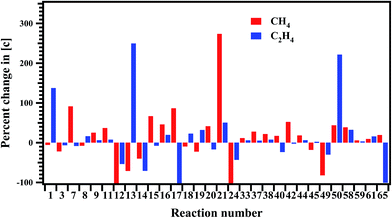Fig. 12 Sensitivity analysis of the proposed reaction scheme carried out at 1347 K for the formation of methane and ethylene.
5.1.2. Ethylene. The other major product is C2H4, which is formed mainly via direct decomposition of MB through a 6 membered transition state (TS1 through reaction (R1)) and a four membered transition state (TS2 through reaction (R2)) (cf. Fig. 2). Concentrations of C2H4 were found to be lower than the concentrations of CH4 by 10% in the studied temperature range. Ethylene is formed through reactions (R1) and (R2) and rate coefficients for these channels are computed theoretically in the present investigation. The activation energies for these two reactions (R1) and (R2) were calculated to be 68 and 106 kcal mol−1 respectively. Therefore, it is obvious that reaction (R1) contribute more to the formation of ethylene when compared to that of (R2).
 MB → C2H4 + CH2C(OH)–O–CH3, k1 = 1.62 × 1013exp(−68.46/RT) s−1 (R1)
 MB → CH3–C(O)–O–CH3 + C2H4, k2 = 2.51 × 1013exp(−106.30/RT) s−1 (R2)

The other main contribution for the formation of ethylene is reaction (R50). The ethylene is formed via β-C–C bond scission of CH2˙–CH2–CH2–C(O)–O–CH3 radical.

 CH2˙–CH2–CH2–C(O)–O–CH3 → C2H4 + CH2˙–C(O)–O–CH3, k50 = 5.25 × 1011(T)0.50exp(−26.59/RT) s−1 (R50)

The reaction between two methyl radicals to form ethylene (R55) is added to the reaction scheme for completeness.

 2CH3˙ → C2H4 + H2, k55 = 9.90 × 1015exp(−32.98/RT) cm3 mol−1 s−1 (R55)

Ethylene reacts with hydrogen atoms and form C2H3˙ radical and H2 through reaction (R65), which is main source for the formation acetylene.

 C2H4 + H → C2H3˙ + H2, k65 = 5.24 × 1014exp(−14.90/RT) cm3 mol−1 s−1 (R65)

The sensitivity analysis of the proposed reaction scheme is carried out for the formation of C2H4 and the results are shown in Fig. 12. Reactions (R1), (R13), and (R50) are very sensitive to the formation of C2H4.

5.1.3. Acetylene. Acetylene is one of the major products obtained in the thermal decomposition of MB. The measured concentration of acetylene is 36% in the present experimental study. The radical CH˙CHC(O)OCH3 can produce C2H2 by C–C(O) bond cleavage. The radical CH˙CH–C(O)–O–CH3 is formed by hydrogen abstraction fromCH2 group of CH2CH–C(O)–O–CH2˙ (this radical in turn is formed via γ-C–C bond scission of methyl butanoate radical through reaction (R43)) through reaction (R46). The other possibility for the formation of acetylene and hydrogen atom is via decomposition of C2H3˙ radicals (R63).
 CH˙CH–C(O)–O–CH3 → C2H2 + CH3–O–C(O)˙, k24 = 5.76 × 1012(T)0.82exp(−38.50/RT) cm3 mol−1 s−1 (R48)
 C2H3˙ → C2H2+ H, k63 = 2.00 × 1014exp(−39.74/RT) s−1 (R63)

The sensitivity analysis is carried out for the formation of C2H2 and the results are shown in Fig. 13. Reactions (R7), (R17) and (R65) are found to be sensitive for the formation of C2H2.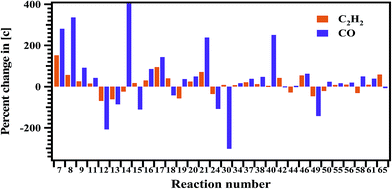Fig. 13 Sensitivity analysis of the proposed reaction scheme carried out at 1347 K for the formation of acetylene and CO.
5.1.4. Minor products. The minor reaction products observed in the experiments are ethane (C2H6), propylene (C3H6), 1,3-butadiene (C4H6) and methyl acrylate. The concentrations of these four products are less than 4%, when compared with the main products. The concentrations of ethane, propylene, 1,3-butadiene seems to be increasing with the temperature in the studied temperature range. The concentration of the other minor product methyl acrylate was observed to be increasing up to 1400 K and beyond this temperature it was found to be decreasing. The most possible channels for the formation of C2H6 is the recombination of two CH3˙ radicals (R54) and reaction between propylene with C2H5˙ radical (R66).
 2CH3˙ → C2H6, k54 = 6.80 × 1014(T)−0.60 cm3 mol−1 s−1 (R54)
 C3H6 + C2H5˙ → C2H6 + C3H5˙, k66 = 2.23(T)3.50exp(−6.64/RT) cm3 mol−1 s−1 (R66)

Propylene is formed directly from C–C(O) bond dissociation of CH3–CH˙–CH2–C(O)–O–CH3 radical (R40). The other channel via which propylene formed is C–H bond scission in n-C3H7˙ radical (R53).

 CH3–CH˙–CH2–C(O)–O–CH3 → C3H6 + CH3–O–C(O)˙, k40 = 4.53 × 1012(T)0.33exp(−34.27/RT) cm3 mol−1 s−1 (R40)
 CH3–CH2–CH2˙ → C3H6+ H, k53 = 4.14 × 1012(T)0.17exp(−35.62/RT) s−1 (R53)

On the other hand, propylene reacts with C2H3˙ and C2H5˙ radicals and form 1,3-butadiene and ethane through reactions (R62) and (R66). The only channel through which 1,3-butadiene can be formed using the present scheme is reaction (R62).

 C3H6 + C2H3˙ → C4H6 + CH3˙, k62 = 7.23 × 1011exp(−5.01/RT) cm3 mol−1 s−1 (R62)

Methyl acrylate is formed by breaking C–C bond of CH3–CH2–CH˙–C(O)–O–CH3 through a β-scission (R42). It is also formed by C–H bond scission at α-position of CH2˙–CH2–C(O)–O–CH3 through reaction (R43). These two reactions are responsible for the formation of methyl acrylate in these experimental conditions.

 CH3–CH2–CH˙–C(O)–O–CH3 → CH2CH–C(O)–O–CH3 + CH3˙, k42 = 1.33 × 1011(T)0.97exp(−34.88/RT) s−1 (R42)
 CH2˙–CH2–C(O)–O–CH3 → CH2CH–C(O)–O–CH3 + H, k43 = 1.53 × 108(T)1.57exp(−38.38/RT) s−1 (R43)

Sensitivity analysis is carried out to check the sensitivity of each reaction towards the formation and decomposition of minor products by varying the rate coefficients of each proposed reaction by a factor of 10. The results are shown in Fig. 14 and 15 in terms of percentage changes in the concentrations with respect to the reaction numbers. The reactions (R7), (R8) and (R24) are sensitive for the formation of C2H6. It shows methyl radicals are key species for the formation of C2H6. Reactions (R14) and (R40) have shown more sensitivity towards the formation of C3H6. The reactions (R7), (R8), (R21), (R44) and (R65) have shown more sensitivity towards the formation of 1,3-butadiene. In the case of methyl acrylate formation, the reactions (R12), (R15), (R42) and (R58) are observed to be more sensitive.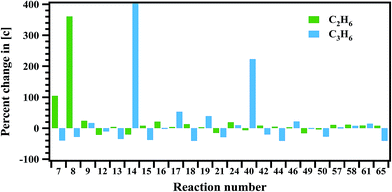Fig. 14 Sensitivity analysis of the proposed reaction scheme carried out at 1347 K for the formation of ethane and propylene.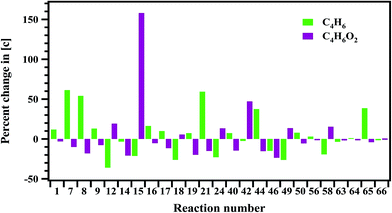Fig. 15 Sensitivity analysis of the proposed reaction scheme carried out at 1347 K for the formation of 1,3-butadiene and methyl acrylate.

It should be noted here that, the agreement between the experimentally measured concentrations and simulated ones was found to be not that satisfactory in case of 1,3-butadiene and methyl acrylate. As the concentrations of these two compounds are very low (1% and 3% respectively), the agreement could not be achieved with the proposed mechanism. As mentioned before, the only channel through which 1,3-butadiene can be formed using the present scheme is reaction (R62).

 C3H6 + C2H3˙ → C4H6 + CH3˙, k62 = 7.23 × 1011exp(−5.01/RT) cm3 mol−1 s−1 (R62)

Although the amount of propylene available for this reaction is plenty, the formation of 1,3-butadiene is subject to the availability of C2H3 radical, whose formation is very minimal. Therefore, the agreement between experiment and simulations is not good, in case of 1,3-butadiene.

Another very important product that we observed in FTIR analysis in the experiments is CO. The contribution for the formation of CO is significant via the reactions (R52), (R58) and (R59).

 CH2˙–CHCO → C2H3˙ + CO, k52 = 3.47 × 1012(T)0.63exp(−27.21/RT) s−1 (R52)
 CH2C(O) + H → CO + CH3˙, k58 = 7.77 × 108(T)1.45exp(−2.78/RT) cm3 mol−1 s−1 (R58)
 CH3–O–C(O)˙ → CO + CH3–O˙, k59 = 8.02 × 1011(T)0.65exp(−21.12/RT) s−1 (R59)

Sensitivity analysis has show reaction (R58) to be more sensitive to the formation of CO.

## 6. Conclusions

In the present investigation, we have reported the rate coefficients for total decomposition of MB in the temperature range of 1229–1427 K, for the first time. The obtained rate coefficient for the total decomposition of MB in the studied temperature range is ktotal (1229–1427 K) = (3.08 ± 1.11) × 1012exp(−(53.6 kcal mol−1 ± 4.7)/RT) s−1. In addition to this we have reported rate coefficients for intramolecular hydrogen transfer reactions ((R1)–(R6)) in thermal decomposition of MB using computational methods. The kinetic data calculated for the unimolecular elimination reactions over the temperature range of 500–2500 K were used to derive the Arrhenius expression: ktheorytotal (500–2500 K) = (9.05 ± 1.91) × 1013exp(−(70.7 kcal mol−1 ± 2.0)/RT) s−1. The computed rate coefficients for the reactions ((R1)–(R6)) were used in kinetic modelling to understand the complete decomposition reaction mechanism. Here, well established theoretical methods are helpful to extrapolate rate coefficients to higher temperatures. The basis of every detailed reaction mechanism in combustion is formed by a set of elementary reactions and its kinetic parameters. The comprehensive modeling of biodiesel combustion requires large amounts of experimental data and extensive investigation on the detailed combustion mechanisms. These rate coefficients obtained in the present study are useful to understand the combustion of large size complex biodiesel molecules by including these elementary reactions in their modelling.

## Acknowledgements

B. R. thanks Council of Scientific and Industrial Research (CSIR), Government of India for funding. A. P. is very grateful to CSIR for providing a research fellowship. A. P. also thank Mr G. Sudhakar and Mr G. Srinivasulu for their help in doing the experiments.

## References

1. M. Besson, P. Gallezot and C. Pinel, Chem. Rev., 2014, 114, 1827–1870 CrossRef CAS PubMed.
2. W. K. Metcalfe, S. Dooley, H. J. Curran, J. M. Simmie, A. M. Al-Nahas and M. V. Navarro, J. Phys. Chem. A, 2007, 111, 4001–4014 CrossRef CAS PubMed.
3. R. Behcet, H. Oktay, A. Cakmak and H. Aydin, Renewable Sustainable Energy Rev., 2015, 46, 157–165 CrossRef CAS PubMed.
4. G. Knothe, C. A. Sharpe and T. W. Ryan, Energy Fuels, 2006, 20, 403–408 CrossRef CAS.
5. M. S. Graboski and R. L. McCormick, Prog. Energy Combust. Sci., 1998, 24, 125–164 CrossRef CAS.
6. K. Bozbas, Renewable Sustainable Energy Rev., 2008, 12, 542–552 CrossRef CAS PubMed.
7. J. M. Simmie, Prog. Energy Combust. Sci., 2003, 29, 599–634 CrossRef CAS.
8. O. Herbinet, W. J. Pitz and C. K. Westbrook, Combust. Flame, 2008, 154, 507–528 CrossRef CAS PubMed.
9. P. Dievart, S. H. Won, S. Dooley, F. L. Dryer and Y. Ju, Combust. Flame, 2012, 159, 1793–1805 CrossRef CAS PubMed.
10. K. Kohse-Hoinghaus, P. Osswald, T. A. Cool, T. Kasper, N. Hansen, F. Qi, C. K. Westbrook and P. R. Westmoreland, Angew. Chem., 2010, 49, 3572–3597 CrossRef PubMed.
11. S. Gail, M. J. Thomson, S. M. Sarathy, S. A. Syed, P. Dagaut, P. Dievart, A. J. Marchese and F. L. Dryer, Proc. Combust. Inst., 2007, 31, 305–311 CrossRef PubMed.
12. A. Farooq, D. F. Davidson, R. K. Hanson, L. K. Huynh and A. Violi, Proc. Combust. Inst., 2009, 32, 247–253 CrossRef CAS PubMed.
13. A. Farooq, W. Ren, K.-Y. Lam, D. F. Davidson, R. K. Hanson and C. K. Westbrook, Combust. Flame, 2012, 159, 3235–3241 CrossRef CAS PubMed.
14. K. C. Lin, J. Y. W. Lai and A. Violi, Fuel, 2012, 92, 16–26 CrossRef CAS PubMed.
15. E. M. Fisher, W. J. Pitz, H. J. Curran and C. K. Westbrook, Proc. Combust. Inst., 2000, 28, 1579–1586 CrossRef CAS.
16. M. H. Hakka, H. Bennadji, J. Biet, M. Yahyaoui, B. Sirjean, V. Warth, L. Coniglio, O. Herbinet, P. A. Glaude, F. Billaud and F. Battin- Leclerc, Int. J. Chem. Kinet., 2010, 42, 226–252 CrossRef CAS PubMed.
17. S. Dooley, H. J. Curran and J. M. Simmie, Combust. Flame, 2008, 153, 2–32 CrossRef CAS PubMed.
18. J. Y. W. Lai, K. C. Lin and A. Violi, Prog. Energy Combust. Sci., 2011, 37, 1–14 CrossRef CAS PubMed.
19. M. A. Ali and A. Violi, J. Org. Chem., 2013, 78, 5898–5908 CrossRef PubMed.
20. G. Sudhakar and B. Rajakumar, J. Chem. Sci., 2014, 126, 897–909 CrossRef CAS.
21. A. G. Gaydon and I. R. Hurle, The Shock Tube in High-Temperature Chemical Physics, Reinhold Publishing, New York, 1963 Search PubMed.
22. A. Lifshitz, C. Tamburu and A. Suslensky, J. Phys. Chem. A, 2006, 110, 11677–11683 CrossRef CAS PubMed.
23. J. A. Manion and I. A. Awan, Proc. Combust. Inst., 2013, 34, 537–545 CrossRef CAS PubMed.
24. M. Akira, Y. Kenji and S. Hiroumi, J. Phys. Chem. A, 2014, 118, 11688–11695 Search PubMed.
25. M. J. Frisch, G. W. Trucks, H. B. Schlegel, G. E. Scuseria, M. A. Robb, J. R. Cheeseman, G. Scalmani, V. Barone, B. Mennucci and G. A. Petersson, Gaussian 09, Revision B.01, Gaussian, Inc., Wallingford, CT, 2010 Search PubMed.
26. Y. Zhao and D. G. Truhlar, Acc. Chem. Res., 2008, 41, 157–167 CrossRef CAS PubMed.
27. Y. Zhao and D. G. Truhlar, Theor. Chem. Acc., 2008, 120, 215–241 CrossRef CAS.
28. G. Srinivasulu and B. Rajakumar, J. Phys. Chem. A, 2013, 117, 4534–4544 CrossRef CAS PubMed.
29. M. Balaganesh, M. R. Dash and B. Rajakumar, J. Phys. Chem. A, 2014, 118, 5272–5278 CrossRef CAS PubMed.
30. M. R. Dash and B. Rajakumar, Atmos. Environ., 2013, 79, 161–171 CrossRef CAS PubMed.
31. I. I. R. Dennington, T. Keith, J. Millam, K. Eppinnett, W. L. Hovell and R. Gilliland, GaussView, version 3.09, Semichem, Inc., Shawnee Mission, KS, 2003 Search PubMed.
32. C. Gonzalez and H. B. Schlegel, J. Chem. Phys., 1989, 90, 2154–2161 CrossRef CAS PubMed.
33. J. Zheng, S. Zhang, B. J. Lynch, J. C. Corchado, Y.-Y. Chuang, P. L. Fast, W.-P. Hu, Y.-P. Liu, G. C. Lynch and K. A. Nguyen, POLYRATE, version 2008, University of Minnesota, Minneapolis,MN, 2009 Search PubMed.
34. J. Zheng, S. Zhang, J. C. Corchado, Y.-Y. Chuang, E. L. Coitiño, B. A. Ellingson and D. G. Truhlar, GAUSSRATE, version 2009-A, University of Minnesota, Minneapolis, MN, 2010 Search PubMed.
35. A. Gonzalez-Lafont, T. N. Truong and D. G. Truhlar, J. Chem. Phys., 1991, 95, 8875–8894 CrossRef CAS PubMed.
36. B. C. Garrett and D. G. Truhlar, J. Phys. Chem., 1979, 83, 1052–1079 CrossRef CAS.
37. B. C. Garrett, D. G. Truhlar, R. S. Grev and A. W. Magnuson, J. Phys. Chem., 1980, 84, 1730–1748 CrossRef CAS.
38. D. H. Lu, T. N. Truong, V. S. Melissas, G. C. Lynch, Y. P. Liu, B. C. Garrett, R. Steckler, A. D. Isaacson, S. N. Rai and G. C. Hancock, Comput. Phys. Commun., 1992, 71, 235–262 CrossRef CAS.
39. Y. P. Liu, G. C. Lynch, T. N. Truong, D. H. Lu, D. G. Truhlar and B. C. Garrett, J. Am. Chem. Soc., 1993, 115, 2408–2415 CrossRef CAS.
40. A. M. El-Nahas, M. V. Navarro, J. M. Simmie, J. W. Bozzelli, H. J. Curran, S. Dooley and W. Metcalfe, J. Phys. Chem. A, 2007, 111, 3727–3739 CrossRef CAS PubMed.
41. A. Lifshitz, C. Tamburu, A. Suslensky and F. Dubnikova, J. Phys. Chem. A, 2004, 108, 3430–3438 CrossRef CAS.
42. L. K. Huynh and A. Violi, J. Org. Chem., 2008, 73, 94–101 CrossRef CAS PubMed.
43. H. J. Curran, Int. J. Chem. Kinet., 2006, 38, 250–275 CrossRef CAS PubMed.
44. A. Lifshitz, C. Tamburu and A. Suslensky, J. Phys. Chem., 1990, 94, 2966–2972 CrossRef CAS.
45. R. D. Kern, H. J. Singh and C. H. Wu, Int. J. Chem. Kinet., 1988, 20, 731–747 CrossRef CAS PubMed.
46. D. F. Davidson, M. D. DiRosa, E. J. Chang, R. K. Hanson and C. T. Bowman, Int. J. Chem. Kinet., 1995, 27, 1179–1196 CrossRef CAS PubMed.
47. J. P. Senosiain, S. J. Klippenstein and J. A. Miller, J. Phys. Chem. A, 2006, 110, 5772–5781 CrossRef CAS PubMed.
48. H. Hippler, F. Striebel and B. Viskolcz, Phys. Chem. Chem. Phys., 2001, 3, 2450–2458 RSC.
49. W. Tsang, J. Phys. Chem. Ref. Data, 1991, 20, 221–273 CrossRef CAS PubMed.
50. D. L. Baulch, C. J. Cobos, R. A. Cox, C. Esser, P. Frank, T. Just, J. A. Kerr, M. J. Pilling, J. Troe, R. W. Walker and J. Warnatz, J. Phys. Chem. Ref. Data, 1992, 21, 411–429 CrossRef CAS PubMed.

### Footnote

Electronic supplementary information (ESI) available. See DOI: 10.1039/c5ra16977a
 This journal is © The Royal Society of Chemistry 2015